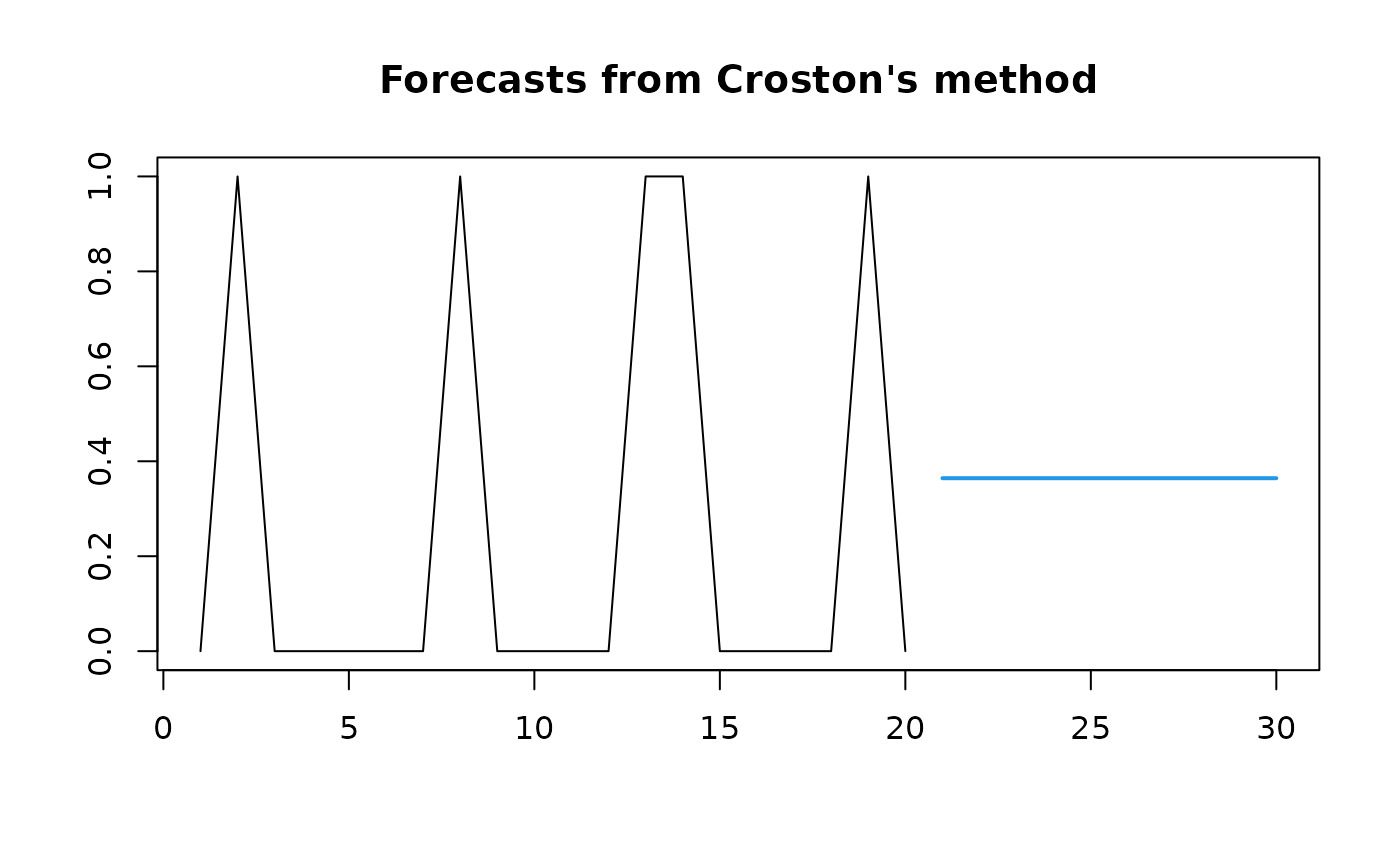Returns forecasts and other information for Croston's forecasts applied to y.

croston(y, h = 10, alpha = 0.1, x = y)

## Arguments

y

a numeric vector or time series of class ts

h

Number of periods for forecasting.

alpha

Value of alpha. Default value is 0.1.

x

Deprecated. Included for backwards compatibility.

## Value

An object of class "forecast" is a list containing at least the following elements:

model

A list containing information about the fitted model. The first element gives the model used for non-zero demands. The second element gives the model used for times between non-zero demands. Both elements are of class forecast.

method

The name of the forecasting method as a character string

mean

Point forecasts as a time series

x

The original time series (either object itself or the time series used to create the model stored as object).

residuals

Residuals from the fitted model. That is y minus fitted values.

fitted

Fitted values (one-step forecasts)

The function summary is used to obtain and print a summary of the results, while the function plot produces a plot of the forecasts.

The generic accessor functions fitted.values and residuals

extract useful features of the value returned by croston and associated functions.

## Details

Based on Croston's (1972) method for intermittent demand forecasting, also described in Shenstone and Hyndman (2005). Croston's method involves using simple exponential smoothing (SES) on the non-zero elements of the time series and a separate application of SES to the times between non-zero elements of the time series. The smoothing parameters of the two applications of SES are assumed to be equal and are denoted by alpha.

Note that prediction intervals are not computed as Croston's method has no underlying stochastic model.

ses.

Rob J Hyndman

## Examples

y <- rpois(20,lambda=.3)
fcast <- croston(y)
plot(fcast)